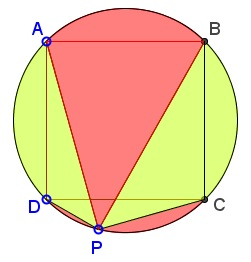# Curious Irrationality in Square

### Problem

Dorin Marghidanu has kindly posted an amazing problem at the CutTheKnotMath facebook page.

Given a square $ABCD\;$ inscribed in a circle and a point $P\;$ on circle arc $CD.$Prove that $\displaystyle\frac{AP+BP}{CP+DP}\;$ is an irrational number.

### Solution 1

Let $s\;$ be the side of the square. Applying Ptolemy's theorem in cyclic quadrilateral $ABPD,\;$we have $AP\cdot BD=BP\cdot AD+DP\cdot AB,\;$ or equivalently, $AP\cdot (s\sqrt{2})=BP\cdot s+DP\cdot s,\;$ i.e.,

(1)

$AP\cdot\sqrt{2}=BP+DP.\;$

Analogously, from the cyclic quadrilateral $ABCP,\;$ we get

(2)

$BP\cdot\sqrt{2}=AP+CP.\;$

Adding relations (1) and (2), we get,

$(\sqrt{2}-1)(AP+BP)=CP+DP,$

which is equivalent $\displaystyle\frac{AP+BP}{CP+DP}=\frac{1}{\sqrt{2}-1}=1+\sqrt{2},\;$ obviously an irrational number.

### Solution 2

W choose $A=-1,\;$ $B=-i\;$ $C=1,\;$ $D=i\;$ and $P=\cos 2t+i\sin 2t,\;$ $\displaystyle 0\lt t \lt\frac{\pi}{4}.$

We get: $AP=2\cos t,\;$ $BP=\sqrt{2}(\cos t+\sin t),\;$ $CP=2\sin t,\;$ and $DP=\sqrt{2}(\cos t-\sin t).\;$ Putting this together,

\displaystyle\begin{align}\frac{AP+BP}{CP+DP}&=\frac{\sin t+(1+\sqrt{2})\cos t}{\cos t+(\sqrt{2}-1)\sin t}\\ &=\sqrt{2}+1, \end{align}

which is clearly not rational.

### Solution 3

We have successively,

$AP=2R\cos\angle CAP;\;CP=2R\sin\angle CAP,\\ BP=2R\cos\angle DBP;\;DP=2R\sin\angle DBP.$

But $\angle CAP+\angle DBP=45^{\circ}.\;$ Thus,

\displaystyle\begin{align} \frac{AP+BP}{CP+DP}&=\frac{\cos\angle CAP+\cos\angle DBP}{\sin\angle CAP+\sin\angle DBP}\\ &=\frac{\cos 22.5^{\circ}\cos\displaystyle\frac{\angle CAP-\angle DBP}{2}}{\sin 22.5^{\circ}\cos\displaystyle\frac{\angle CAP-\angle DBP}{2}}\\ &=\cot 22.5^{\circ}. \end{align}

And $\cot 22^{\circ}\;$ is irrational.

### Solution 4

Let $PP'\;$ be a diameter of $(ABCD).\;$ Complement the diagram:Then observe four right isosceles triangles. We have, $AP=AF+FP=CP+DP\sqrt{2}\;$ and $BP+BE+EP=DP+CP\sqrt{2},\;$ implying $AP+BP=(\sqrt{2}+1)(CP+DP).$

### Solution 5

Triangles $ACP\;$ and $BDP\;$ are right.Assuming $AB=1,\;$ $AC=BD=\sqrt{2}.\;$ With $\alpha=\angle CAP\;$ and $\beta=\angle DBP,\;$ we have $\alpha+\beta=45^{\circ}\;$ and

$AP=\sqrt{2}\cos\alpha;\;CP=\sqrt{2}\sin\alpha,\\ BP=\sqrt{2}\cos\beta;\;DP=\sqrt{2}\sin\beta.$

It follows that

\displaystyle\begin{align} \frac{AP+BP}{CP+DP} &= \frac{\cos\alpha+\cos (45^{\circ}-\alpha)}{\sin\alpha+\sin (45^{\circ}-\alpha)}\\ &=\frac{\cos\alpha +\cos 45^{\circ}\cos\alpha+\sin 45^{\circ}\sin\alpha}{\sin\alpha+\sin 45^{\circ}\cos\alpha-\cos 45^{\circ}\sin\alpha}\\ &=\frac(1+\frac{\sqrt{2}}{2})\cos\alpha +\frac{\sqrt{2}}{2}\sin\alpha}(1-\frac{\sqrt{2}}{2})\sin\alpha +\frac{\sqrt{2}}{2}\cos\alpha}\\ &=\frac{(\sqrt{2}+1)\cos\alpha +\sin\alpha}(\sqrt{2}-1)\sin\alpha +\cos\alpha}\\ &=\sqrt{2}+1. \end{align

### Solution 6Assuming the side of the square equals $1,\;$ apply the Law of Sines in triangles $ADP\;$ and $BCP\;$ to obtain

$AP=\sqrt{2}\sin (135^{\circ}-\beta );\;DP=\sqrt{2}\sin\beta,\\ BP=\sqrt{2}\sin (135^{\circ}-\alpha);\;CP=\sqrt{2}\sin\alpha$

which leads to a derivation similar to that of Solution 5.

### Solution 7

Let $PP'\;$ be the chord parallel to $AD.\;$ Reflect $P'\;$ in $AB\;$ to get the orthocenter $H\;$ of triangle $PAB.$ Note that $AH=AP'=DP\;$ and $BH=BP'=CP.$There are four isosceles right-angled triangles: $AH_bH,\;$ $BH_aH,\;$ $AH_aP,\;$ and $BH_bP.\;$ From these:

$\displaystyle AP=AH_b+H_bP=\frac{1}{\sqrt{2}}DP+\frac{1}{\sqrt{2}}BP,\\ \displaystyle BP=BH_a+H_aP=\frac{1}{\sqrt{2}}CP+\frac{1}{\sqrt{2}}AP,$

implying $(AP+BP)(\sqrt{2}-1) = CP + DP\;$ which is the same as $AP+BP = (CP + DP)(\sqrt{2}+1).$

### Solution 8

As it was shown elsewhere, the ratio $\displaystyle\frac{AP+BP}{CP+DP}\;$ remains constant, regardless of the position of $P\;$ on arc $CD.\;$ Thus we consider an extreme case where, e.g., $P=D.$ Then we have

$\displaystyle\frac{AP+BP}{CP+DP}=\frac{AD+BD}{0+CD}=\sqrt{2}+1.$

### Acknowledgment

Solution 1 is by Dorin Marghidanu; Solution 2 by Leo Giugiuc and Dan Sitaru; Solution 3 by By Michael Anderson from the group "Mathematics"; Solution 4 by Trung Vu; Solution 7 is by Gregoire Nicollier.

### Extra

Daniel Liu has observed that the configuration at hand has another pleasing property:

The areas in the diagram below, marked yellow and red, are equal:We'll show that the yellow area fills half the circle. Indeed, the square cuts off the circle four equal circular segments - two of them are yellow. For the triangle areas, $[\Delta ADP]+[\Delta BCP]=\frac{1}{2}[ABCD].$

Tim Robinson has noted that $P\;$ need not lie on the circle $(ABCD)\;$ for Daniel's observation to remain true.

In addition, we also have $\displaystyle\frac{CP-DP}{BP-AP}=\sqrt{2}+1,\;$ exactly as irrational as $\displaystyle\frac{AP+BP}{CP+DP}.$